# The probability depends on the order

## Conditional probability

If you roll the dice several times, the probability of throwing a certain number between 1 and 6 does not depend on the previous result.
Each throw happens independently of the previous one.
If, on the other hand, balls are drawn one after the other from an urn that contains, for example, several balls with two different colors, without putting them back, then the probability of a certain result often depends on the previous result.
In this case one speaks of a conditional probability.

### Introductory example

One urn contains 100 balls.
70 balls are made of wood and 30 balls are made of plastic.
25 of the wooden balls are painted red and 45 are green.
10 of the plastic balls are red and 20 are green.
The following events are defined:The spheres have two characteristics, each with two characteristics.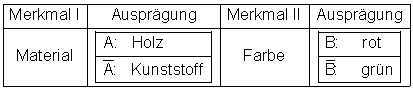This fact can be in a Four-field table being represented:A ball is drawn at random from the urn.

The following probabilities can be calculated directly with the data on the board: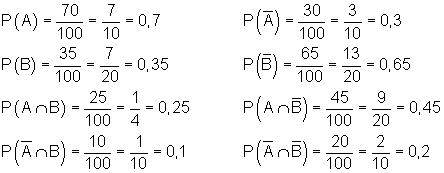The associated four-field table: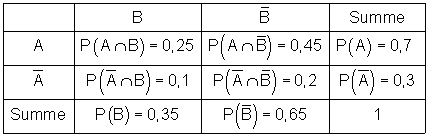Someone pulls a ball and feels with their hand that it is a plastic ball.
So what is the probability that the ball in his hand is green?
That's not the likelihood of pulling a green plastic ball.
The probability sought cannot be read from the four-field table.

This question should now be clarified with an event tree.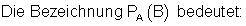The probability of B under the condition that A has already occurred.
We call this probability a conditional probability.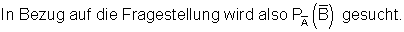In words:
What is the probability of having drawn a green ball, if you know that the drawn ball is made of plastic?
A probability is sought that depends on a condition.
In this case the condition is: The drawn ball is made of plastic.

The path multiplication rule is used to calculate the probabilities that are still missing in the tree diagram:The rule by which the conditional probability is calculated goes back to the English mathematician Thomas Bayes (1702 - 1761) and is therefore also called Bayes’s rule or Bayes’s theorem.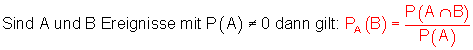So if you know that the drawn ball is made of plastic, then the probability that it is green is 2/3. The probability of pulling a green plastic ball, however, is 0.2.

### A slightly different approach:

An urn contains 3 green and 2 red balls.
Two balls are drawn one after the other without replacing.
Four events are defined:
A: Green is drawn on move 1.
B: Green is drawn on move 2.
C: Green is drawn on the first and second moves.
D: Green on the second move on the condition that green was already drawn on the first move.

The probabilities of all events are to be determined.

A Tree diagram with the path probabilities illustrates the relationship.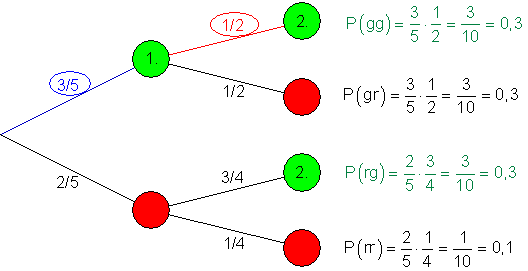The value of P (D) was determined as follows:
Provided that green was drawn on move 1, you know that there are still 2 green and 2 red balls in the urn.
The probability for green on move 2 is then 1/2.
For the probability of D (green in the 2nd move) under the condition that A (green in the 1st move) has already occurred, one chooses the designation P (D) = PA.(B).The excerpt from the is used for further investigation Path diagram, in which PA.(B) occurs.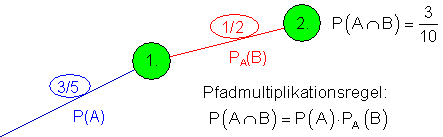Is according to the probability PA.(B) asked, the above equation can be transformed as follows: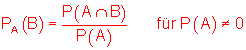PA.(B) is the probability of B under the condition that A has already occurred.
We review this law with the available results:From the urn attempt (multiple draws without replacing) it is clear that the probability of the next drawing depends on the previous one.
In such a case, the events are said to be interdependent.

### Independence from events

In the case of an urn attempt (multiple draws with replacement), the initial condition is always restored so that the probability for the next drawing is the same as for the first.
In such a case, the events are said to be independent of one another.

An urn contains 3 green and 2 red balls.
Two balls are drawn one after the other with replacement.
Four events are defined:
A: Green is drawn on move 1.
B: Green is drawn on move 2.
C: Green is drawn on the first and second moves.
D: Green on the second move on the condition that green was already drawn on the first move.

The tree diagram with the associated path probabilities: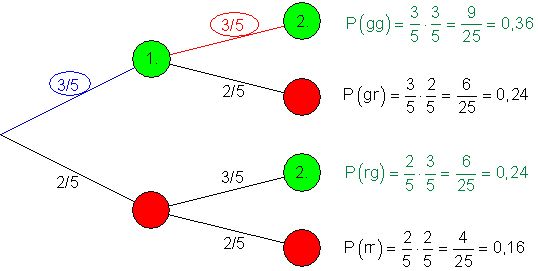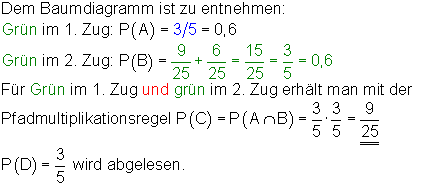The probability of pulling a green ball always remains the same, since after each move the starting situation is restored by replacing the ball.
The probability for green in the 2nd move under the condition that green was already drawn in the 1st move is P (D) = PA.(B).

An excerpt from the tree diagram: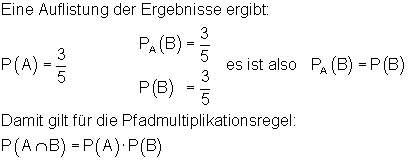Does PA.(B) = P (B), then the occurrence of the event A does not affect the probability of B.
It is said that events A and B are independent of each other.

### Definition: Independent events:

The event B is called independent of the event A if the occurrence of A does not influence the probability of the occurrence of B.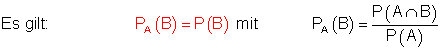Example: voting with replacement.

### Note:

Proceed as follows to prove the independence of two events A and B: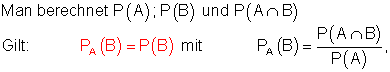then the events A and B are independent of each other, otherwise the events A and B are dependent on each other. In the tree diagram you can recognize the independence of events from the fact that the subtrees are the same in the 2nd level. On the other hand, if they are different, then the events are interdependent.

### example 1

The side faces of an ideal cube are colored as follows.
Two side faces with the paint red and two with the color green. A side face with the color black and one with the color blue.
The dice is thrown twice. The following events are defined:
A: The color appears on the first throw red or black.
B: The color appears on the second throw green or blue.
Investigate whether events A and B are independent of each other.Two events A and B are independent of each other if:Events A and B are independent of each other.

The relationships are now to be examined more closely on a 4-field table and the associated tree diagram. Are known:The remaining values ​​for the 4-field table can be calculated from this.For the tree diagram, all conditional probabilities are now required, which can easily be calculated with the values ​​of the 4-field table.The comparison with the 4-field table shows that all events independently are from each other, because it applies.The independence of the events from one another can also be seen from the fact that the subtrees are the same in the 2nd level of the tree diagram.

### Example 2

A cube in the form of a triangular pyramid has 4 faces of the same size with the numbers 1; 2; 3; 4 (dice of 4).
The dice is thrown twice. The following events are defined:
A: The number 1 or 2 appears on the 1st throw and the number 1 appears on the 2nd throw; 2 or 4.
B: The number on the 2nd throw is different from the number on the 1st throw.
Investigate whether events A and B are independent of each other.Two events A and B are independent of one another if: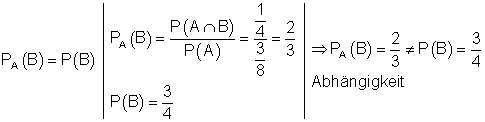Events A and B are interdependent.

The relationships are now to be examined more closely on a 4-field table and the associated tree diagram. Are known:The remaining values ​​for the 4-field table can be calculated from this.For the tree diagram, all conditional probabilities are now required, which can easily be calculated with the values ​​of the 4-field table.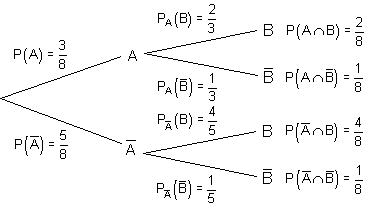The comparison with the 4-field table shows that all events dependent are from each other, because it applies.The interdependence of the events can also be seen from the fact that the subtrees in the 2nd level of the tree diagram are not equal.

### Example 3

A survey of school students' eating habits found that 45% of all students enjoy eating chocolate.
55% of all students prefer other sweets.
60% of all students said they had siblings.
27% of students have siblings and enjoy eating chocolate.

A chocolate manufacturer is interested in whether schoolchildren with siblings have a particular fondness for chocolate.
In other words, does the fact that a student has siblings affect their preference for chocolate?

The survey data can be presented in a four-field table:The associated events are:
A: The student has siblings.
B: The student likes to eat chocolate.

Checking for dependency:The events are independent of each other.
This means whether or not a student has siblings does not affect their preference for chocolate.

The relationships should now be examined more closely using the associated tree diagram. For the tree diagram, all conditional probabilities are now required, which can be easily calculated with the values ​​of the 4-field table.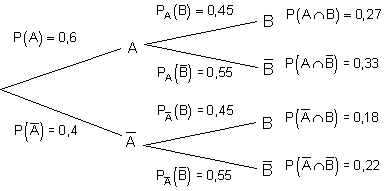The comparison with the 4-field table shows that all events independently are from each other, because it applies.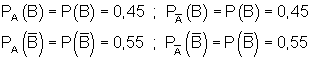The independence of the events from one another can also be seen from the fact that the subtrees are the same in the 2nd level of the tree diagram.

### Example 4 with four-field table and tree diagram

A vocational college has 1000 students. The following four-field table shows how the cell phones are distributed among the students.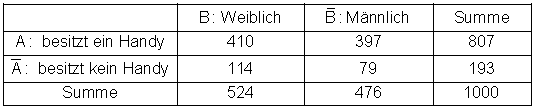a) Calculate the relative frequencies and enter them in a new four-field table.
b) Use the relationship between a four-field table and the tree diagrams to draw the trees.
c) Calculate all conditional probabilities and enter them in the tree diagrams.
d) One is chosen at random from all the students.
1) What is the probability that the person does not have a cell phone?
2) What is the probability that the person is female?
3) If a selected person does not have a cell phone, what is the probability that they are male?
4) If a selected person is female, what is the likelihood of having a cell phone?
5) Is there a connection between gender and cell phone ownership?

### Solutions: a)

b)c) Calculation of the conditional probabilities: Read the probabilities from the four-field table and assign them to the events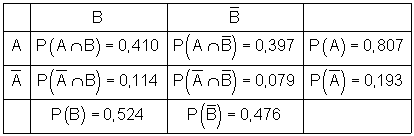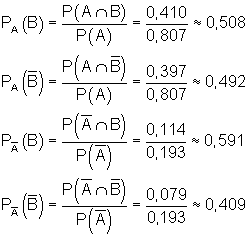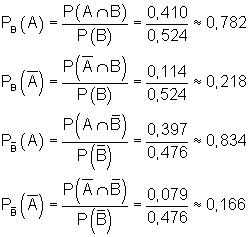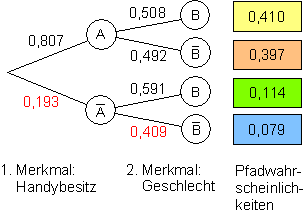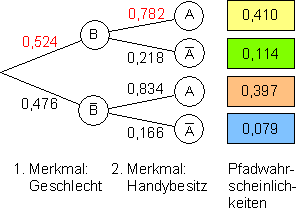d) The probabilities sought can now be read directly from the tree diagrams.
1.)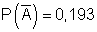A randomly selected person has no cell phone with a probability of 0.193.
2.)A randomly selected person is female with a probability of 0.524.
3.)A randomly selected person who is known to have no cell phone is male with a probability of 0.409.
4.)A randomly selected person who is known to be female has a cell phone with a probability of 0.782.
5.) Checking for dependency. (Relationship between gender and cell phone ownership)Otherwise the events are interdependent.
With the results already available it can be shown: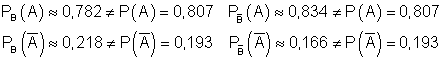This means that in all cases there is a dependency between gender and cell phone ownership.

The dependency of the events can be read directly from the tree diagrams, because the subtrees of the 2nd level are different.
Once you have understood the connection between a four-field table and the tree diagrams, such tasks can also be solved with less effort. The following example should show this.

### Example 5

Many internet users complain about spam mail.
Let us assume that the word “Viagra” appears in 1% of the good and 40% of the spam mails.
In addition, 10% of the mails are good and 90% are spam.
What is the probability that an email that you know contains the word “Viagra” is spam?Set up the four-field table with the given data.
The% values ​​correspond to relative frequencies (probabilities).

90% spam means total spam = 0.9
10% good mails means total good mails = 0.1
40% of spam emails with Viagra means 0.9 x 0.4 = 0.36
1% of good emails with Viagra means = 0.1 x 0.01 = 0.001
The remaining values ​​can be calculated because the sums are known.

 Spam without Viagra: Good mail without Viagra: Sum of all emails with Viagra: Sum of all emails without Viagra: 0,9 – 0,36 = 0,54 0,1 – 0,001 = 0,099 0,36 + 0,001 = 0,361 0,54 + 0,099 = 0,639

The four-field table is now completed with these values.

What is the probability that an email containing “Viagra” is spam?
We are looking for the probability of B under the condition that A has occurred.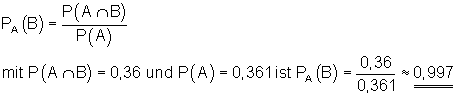This means that in 99.7% of all cases an email containing Viagra is spam.

### Exercise:

The table shows women and men in a company, divided into smokers and non-smokers.a) Calculate the probability of meeting a person who is a smoker.
b) Calculate the probability of meeting a woman.
c) Calculate the probability of meeting a smoker.
d) If you meet a woman, what is the probability that she is a smoker?
e) Check whether events A and B are interdependent.

Solution below

### Conditional probability:

For the probability of occurrence of event B under the condition that event A has already occurred, the following applies:### Independent events:

The event B is called independent of the event A if the occurrence of A does not influence the probability of the occurrence of B.### Relationship between four-field table and tree diagram

Four-field table

Tree diagramIf you swap the order of the observed events in a tree diagram, you get that reverse or inverse Tree diagram. The probabilities at the path ends are the same in both tree diagrams except for the order. The path probabilities and thus also the conditional probabilities generally differ from one another. They relate to different events and therefore also to different subpopulations. But please note: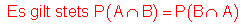### Inverted tree diagram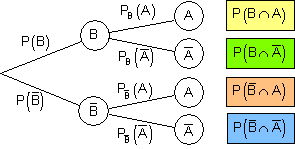### Four-field table and tree diagram with stochastic independence

In the case of stochastically independent events A and B, the first field of the four-field table stands for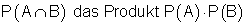.

The same applies to the other fields in accordance with a multiplication table.
In the tree diagram, the independence of events can be recognized by the fact that the subtrees are the same in the 2nd level.

### Solution to exercise:

The table shows women and men in a company, divided into smokers and non-smokers.a) Calculate the likelihood of meeting someone who is a smoker.
b) Calculate the probability of meeting a woman.
c) Calculate the probability of meeting a smoker.
d) You meet a woman, what is the probability that she is a smoker?
e) Check whether events A and B are interdependent.

Solution: In order to be able to determine the probabilities, we need the relative frequencies of the events. In the previous example there were rounding errors. In order to avoid this as possible, the relative frequencies and the associated probabilities should be shown in fractions.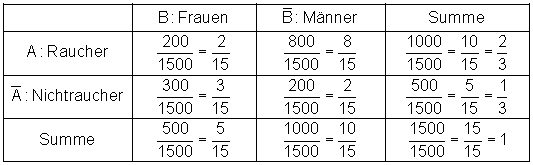a) The probability of meeting a person who is a smoker isb) The probability of meeting a woman is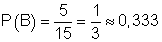c) The probability of meeting a smoker is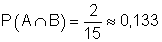d) The probability that a woman encountered is a smoker ise) Two events A and B are independent of each other if: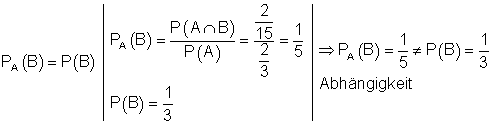Events A and B are interdependent.